# Time Dilation and Length Contraction

This is part of the HSC Physics course under the topic Light and Special Relativity

### HSC Physics Syllabus

• investigate the evidence, from Einstein’s thought experiments and subsequent experimental validation, for time dilation t=t_o/sqrt((1-v^2/c^2)) and length contraction l=l_osqrt((1-v^2/c^2)), and analyse quantitatively situations in which these are observed, for example:

• observations of cosmic-origin muons at the Earth’s surface
• atomic clocks (Hafele–Keating experiment)
• evidence from particle accelerators
• evidence from cosmological studies

### Experimental Evidence for Time Dilation & Length Contraction

Time dilation and length contraction are not postulates but the implication of the constant nature of light's speed, as proposed by Einstein.

### Simultaneity

• The concept of simultaneity – occurrence of simultaneous events is not absolute. This means two simultaneous events may be so for one observer, they may not occur at the same time for another.
• A simple thought experiment can be used to clarify this understanding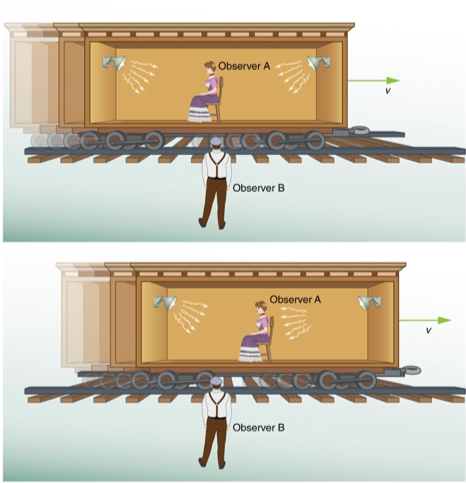Figure 1: Thought experiment demonstrating the effect of special relativity on simultaneity. Credit to Lumen Learning.

Observer A is sitting on a train carriage moving at a substantially fast velocity v. Two sources of light are located at the front and back end of the carriage respectively, equidistant from observer A. When they are turned on simultaneously, observer A receives both stimuli at the same time as the distance between the source and observer remains the same.

Observer B, however, does not observe the sources of light simultaneously. This is because during the time light travels from their sources to observer B, the carriage would have displaced by a certain distance (to the right). This displacement causes the distance between sources of light to observer B to become different.

By Einstein’s second postulate of special relativity (speed of light is constant), the light emitted from the rear end of the carriage will take a shorter time to reach observer B. Light emitted from the front end of the carriage will take a longer time to reach observer B.

### What is Time Dilation?

• The non-absolute nature of simultaneity gives rise to time dilation (one of the three relativistic consequences of the constancy of light)
• The understanding of time dilation can be consolidated and quantitatively analysed using another thought experiment.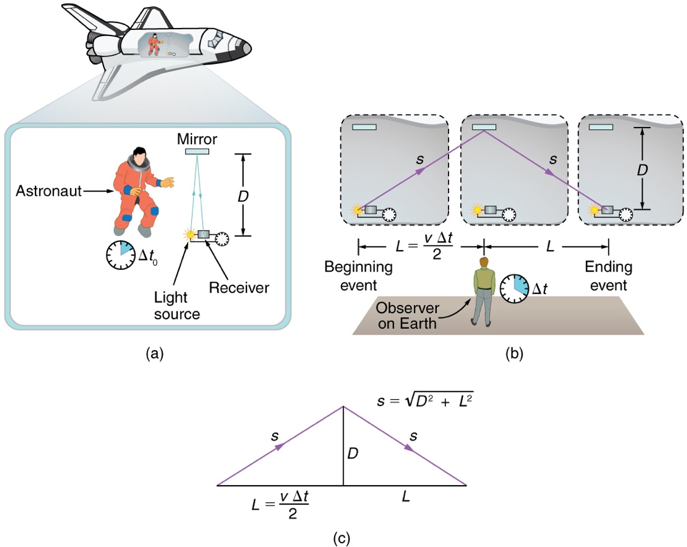Figure 2: Thought experiment demonstrating the effect of special relativity on time. Time becomes dilated (longer) when an object is travelling at relativistic speeds as measured by a stationary observer. Credit to Lumen Learning.

Suppose an astronaut wants to measure the time taken for a beam of light to travel back and forth the width (D) of the spaceship, by reflecting off a mirror. The time taken (t0) would be the total distance divided by the velocity of light:

t_o=(2D)/c

However, to an observer outside the spacecraft, the distance travelled by light is longer. To calculate this distance, we need to use Pythagoras’ theorem to obtain:

s=sqrt(D^2+((vt)/2)^2)

Since the distance travelled observed by the person outside the spacecraft is 2s, the time taken for light to travel to and from the mirror in the cabin is:

t=2s/c

By substituting with the expression we derived previously:

t=(2sqrt(D^2+((vt)/2)^2))/c
Square both sides:
t^2=(4(D^2+((vt)/2)^2))/c^2
Simplify:
t^2=(4D^2)/c^2+(v^2*t^2)/c^2

Since the time (t0) taken by light observed by the astronaut inside the spacecraft is:

t_0=(2D)/c

Therefore by substituting:

t^2=t_o^2+(v^2*t^2)/c^2

Rearrange:

t^2-(v^2*t^2)/c^2=t_o^2

Factorise:

t^2(1-v^2/c^2)=t_o^2

Make t2 the subject of equation:

t^2=t_o^2(1-v^2/c^2)

Finally, square root both sides of equation:

t=t_o/sqrt(1-v^2/c^2)

• where t is the time observed by an observer with relative motion to the event being observed. E.g. person outside the spacecraft.
• t0 is the proper time observed by an observer at rest relative to the event being observed. E.g. astronaut moving at the same velocity as the spacecraft.

### Length Contraction

Distance depends on the observer’s relative motion. Since distance is the product of time and speed, shorter time entails a shorter distance covered.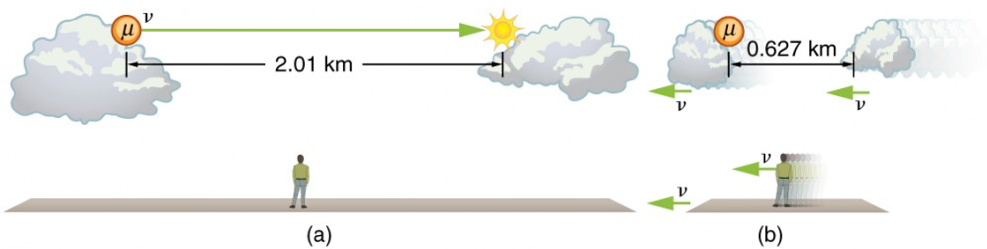The velocity of a particular object relative to an observer at rest is the proper length divided by the dilated time. Proper length l0 is the distance between two points measured by an observer who is at rest relative to both of the points.

v=l_o/t

As such, for an observer who is at rest relative to the moving object (moving at the same speed), velocity is defined as:

v=l/t_o

In this case, the relative velocity in both reference frames are equal:

l_o/t=l/t_o

Make l the subject of equation:

l=l_o(t_o/t)

Since:

t_o/t=sqrt((1-v^2/c^2))

By substitution:

l=l_osqrt((1-v^2/c^2))

• where is the length measured by an observer moving at the same speed as the object travelling the observed length/distance.
• lo is the length measured by an observed at rest relative to the start and end points of the observed length/distance

An object travelling a relativistic velocity experiences length contraction in the dimension of its movement. Its length does not contract in all dimensions.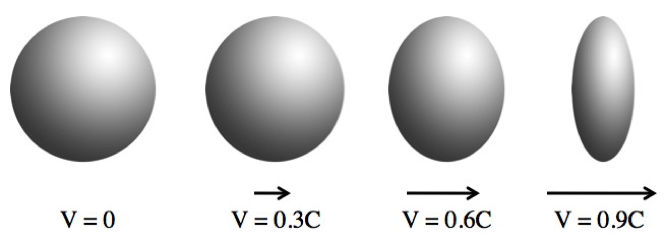### Limitation of Special Relativity

Relativistic effects due to special relativity have two limitations:

• These effects are negligible when a frame of reference is not moving at a relativistic speed.
• Relativistic effects can occur in non-inertial frames of reference but in these scenarios, they are not only attributed to special relativity. Effects due to general relativity must be considered in non-inertial frames of reference. As a result, only relativistic effects in inertial frames of reference are entirely due to special relativity.

## Evidence for Time Dilation & Length Contraction

### Experiments Involving Muons

Muons are cosmic ray particles formed in Earth’s atmosphere. When formed, they travel at velocities near the speed of light towards Earth's surface. However, due to their extremely short half life, majority of muons would decay before reaching the surface.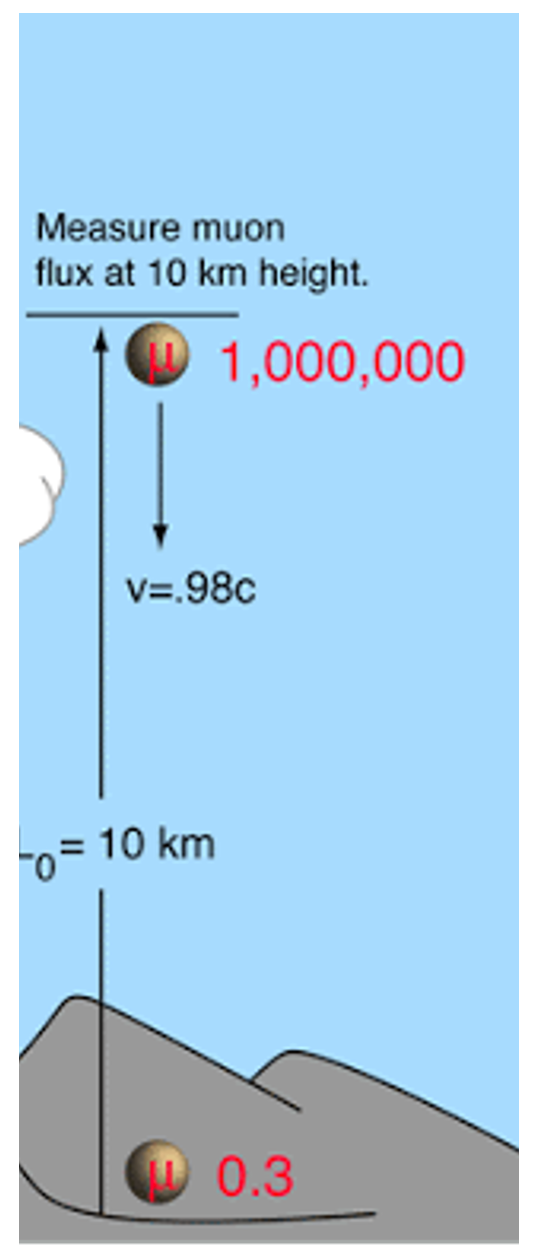In an experiment conducted at Mount Washington, quantities of muons were measured at the summit and bottom of the mountain. These numbers were then compared.

A greater proportion of muons was detected at sea level compared to what was originally predicted by considering the muons' average velocity and half-life at rest (1.5 µs)

Evidence for Time Dilation

This observation can be accounted for using time dilation. When muons are moving near the speed of light (0.98c), their half-life is increased when measured by a stationary observer on Earth. This dilated half-life as observed by an Earth-bound observer allows muons to reach the surface of Earth before decaying.

Evidence for Length Contraction

This observation can also be accounted for using length contraction. In the  muons' frame of reference, the distance between the top of Mount Washington and sea level becomes shorter due to length contraction.

Therefore, in the same amount of lifetime before decaying, more muons can reach Earth's surface.

### Hafele-Keating Experiment

In 1971, Joseph Hafele and Richard Keating deonstrated time dilation using  caesium-beam atomic clocks.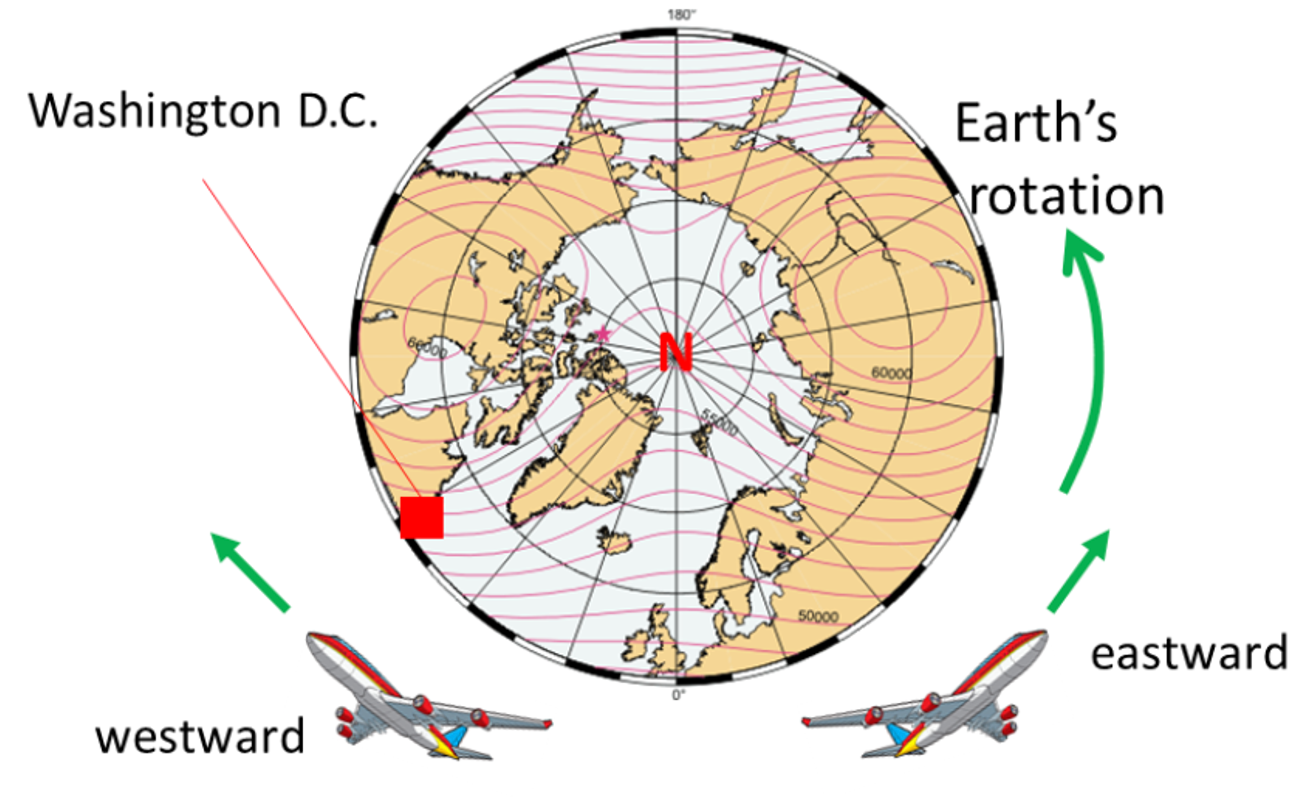Twelve clocks were used in total. Four clocks were flown on a plane in an eastward direction, four were flown in a westward direction, and the last four remained on Earth. After being flown twice around Earth, the times on the three groups of clocks were compared.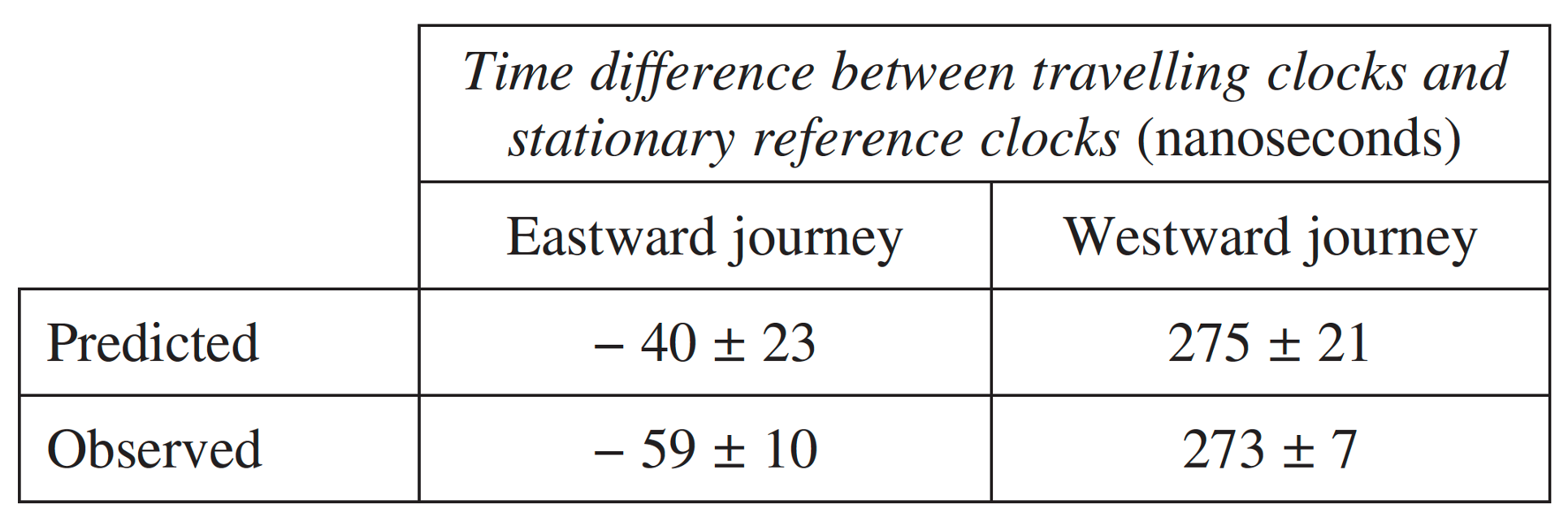The atomic clocks, flown eastward, moved slower (as observed from an Earth-bound observer) and consequently 'lost time'. In other words, a shorter time elapsed on these clocks compared to those on Earth.

In contrast, the atomic clocks flown westward, moved faster and gained time. In other words, a longer time elapsed on these clocks compared to those on Earth.

This experiment is not the best to discuss as evidence for special relativity as the effects of general relativity also affects the experimental data. However, after the effect of general relativity are accounted for, the observed differences in time were consistent with predictions made using time dilation equations.

### Evidence of Special Relativity from Particle Accelerators

In 2014, an experiment was conducted to demonstrate time dilation using lithium ions travelling at 0.338c in a particle accelerator.

The time interval between excitation of electrons in lithium ions and their return to ground state was measured when lithium ions are travelling at 0.338c and at rest.

Physicists found that the interval was longer for moving lithium ions compared to those at rest, as measured by a stationary observer in the laboratory. This difference in time was consistent with time dilation.

Previous section: Evidence for Einstein's Postulates

Next section: Relativistic Momentum and Energy-Mass Equivalence